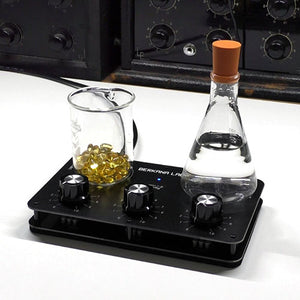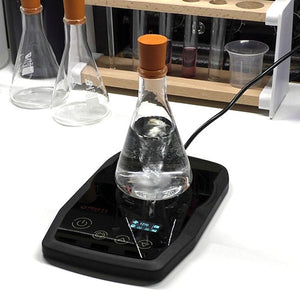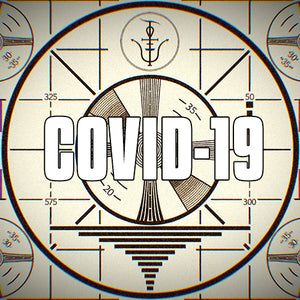# Balance Rate

April 25, 2018Balancing a rate or a compensatory rate is a method of inverting a radionic rate to balance out its effects. If someone had a negative reaction to penicillin for instance you could create a balance rate for it by using the maximum of the rate base, in our case 10, and subtracting each digit from it. Lets break this down and use penicillin as the example.

Penicillin 719998999

10 – 7 = 3
10 – 1 = 9
10 – 9 = 1
10 – 9 = 1
10 – 9 = 1
10 – 8 = 2
10 – 9 = 1
10 – 9 = 1
10 – 9 = 1

This gives us a penicillin balance rate of 391112111

This compensatory rate then acts as a mirror signal out of phase of the original intention. It is then broadcast to the witness or imprinted to water and taken as a radionic remedy.

This out of phase rate acts on the cause of the imbalance, creating a destructive interference pattern due to the two phases cancelling each other.

You can apply this balance rate technique to any sample you have analysed yourself and it will subtly work to balance out any negative reactions.

If the rate your were intending to balance included a zero in its string of digits, this is often left untouched, you would not normally turn 0 into 10 for a balance/complimentary rate.

Remember a zero is often denoted by (0) or 123.045 to distinguish it from 10 so as not to confuse it with 1, this is usually highlighted when a 0 follows 1.

When to Balance?

A radionic practitioner will usually determine the type of rate to use during initial dowsing. Failing that the standard rate is used, and if no improvement is noted during the broadcast, then a complimentary or balance rate can be used.April 16, 2020

In this post I will take you through the process of a very basic radionic substance copying procedure into a simple water remedy, this is an ideal introduction for new users and only has a few steps.